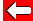# Solving Algebra

To solve problems in algebra, all we need to do is get all the numbers on one side of the equals sign. Let's try: y + 5 = 12

We can add or subtract numbers from either side of the equation as long as we make sure we do the same to each side. Let's subtract 5:

y + 5 - 5 = 12 - 5

Taking 5 off both sides simplifies things on the left of the equals sign (since + 5 - 5 means no change!): y = 12 - 5

So the answer is y = 7Go back a pageMaths MenuGo to next page